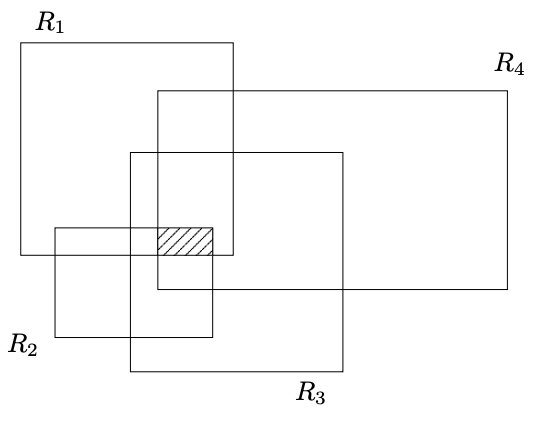시간 제한 메모리 제한 제출 정답 맞은 사람 정답 비율
2 초 512 MB 0 0 0 0.000%

## 문제

Given a set of rectangles {R1, R2, · · · , Rn}, compute the area of their common intersection. i.e.,

Area (R1 ∩ R2 ∩ · · · ∩ Rn)

The edges of the rectangles R1, R2, · · · , Rn, are either vertical or horizontal lines.

For example, the intersection of 4 rectangles, R1, R2, R3, and R4, in the following figure is the shaded rectangle.## 입력

T.IN The first line specifies the number of rectangles n, where 1 < n < 1,000. Since the sides of the rectangles are parallel to the x and y axes, each rectangle is bounded by the lines x = x1, x = x2, y = y1 and y = y2 and each subsequent line of the input thus specifies one rectangle in the following format:

x1 x2 y1 y2

such that 0 ≤ x1 < x2 ≤ 10,000 and 0 ≤ y1 < y2 ≤ 10,000, and x1, x2, y1 and y2 are int

## 출력

The output contains the area of the intersection of all the rectangles.

## 예제 입력 1

2
0 2 0 2
1 3 1 3


## 예제 출력 1

1


## 예제 입력 2

3
1 4 1 8
0 2 0 5
10 15 22 35


## 예제 출력 2

0